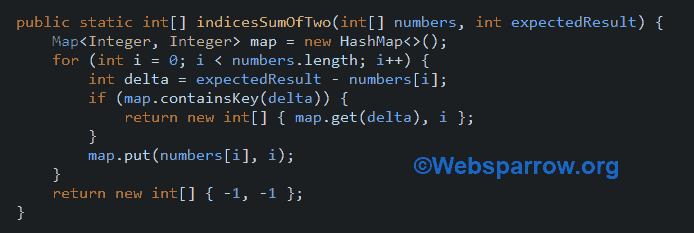# Java- Find the index of the two numbers in the array whose sum is equal to a given numberSometimes interviewer wants to check the candidate’s logical ability and the approach taken to solve the challenge/problem using Java.

Question:  Return the indices of the two numbers such that they add up to a specific target of given an array of integers.

Example:

``````Given nums = [2, 5, 11, 8, 99, 4], target = 9,

Because nums + nums = 5 + 4 = 9,

return [1,5].``````

The above problem can be solved in two ways:

1. Efficient Solution

By using some data structure we can do it more efficiently. Here create a `HashMap` and loop through the length of an array, calculate the delta of target & current number (`delta = target - nums[i]`), and check if the delta is available then return it.

``````public static int[] indicesSumOfTwo(int[] numbers, int expectedResult) {

Map<Integer, Integer> map = new HashMap<>();
for (int i = 0; i < numbers.length; i++) {
int delta = expectedResult - numbers[i];
if (map.containsKey(delta)) {
return new int[] { map.get(delta), i };
}
map.put(numbers[i], i);
}
return new int[] { -1, -1 };
}``````

2. Brute Force Solution

A brute force/native solution is not recommended.  In this, create an outer and inner loop and check if `nums[i] + nums[j] == target`, return the i & j.

``````public static int[] indicesSumOfTwoNativeSolution(int[] numbers, int expectedResult) {

for (int i = 0; i < numbers.length; i++) {
for (int j = 0; j < numbers.length; j++) {
if (numbers[i] + numbers[j] == expectedResult) {
return new int[] { i, j };
}
}
}
return new int[] { -1, -1 };
}``````

Note: We have to handle the exception if indices of the two numbers is not equal to a specific target. In this example, we have returned the -1, -1.

See the complete example.

SumOfTwo.java
``````package org.websparrow.interview;

import java.util.HashMap;
import java.util.Map;

public class SumOfTwo {

public static void main(String[] args) {

int[] numbers = { 2, 5, 11, 8, 99, 4 };
int expectedResult = 9;

int[] result = indicesSumOfTwo(numbers, expectedResult);

System.out.println("(Efficient Solution) Indices: " + result
+ " and " + result);

int[] result1 = indicesSumOfTwoNativeSolution(numbers, expectedResult);

System.out.println("(Native Solution) Indices: " + result1 + " and "
+ result1);

}

// Efficient approach
public static int[] indicesSumOfTwo(int[] numbers, int expectedResult) {

Map<Integer, Integer> map = new HashMap<>();

for (int i = 0; i < numbers.length; i++) {

int delta = expectedResult - numbers[i];

if (map.containsKey(delta)) {

return new int[] { map.get(delta), i };
}
map.put(numbers[i], i);
}
return new int[] { -1, -1 };
}

// Brute force approach
public static int[] indicesSumOfTwoNativeSolution(int[] numbers,
int expectedResult) {

for (int i = 0; i < numbers.length; i++) {

for (int j = 0; j < numbers.length; j++) {
if (numbers[i] + numbers[j] == expectedResult) {

return new int[] { i, j };
}
}
}
return new int[] { -1, -1 };
}

}``````

Output

``````(Efficient Solution) Indices: 1 and 5
(Native Solution) Indices: 1 and 5``````

### References

#### Similar Posts